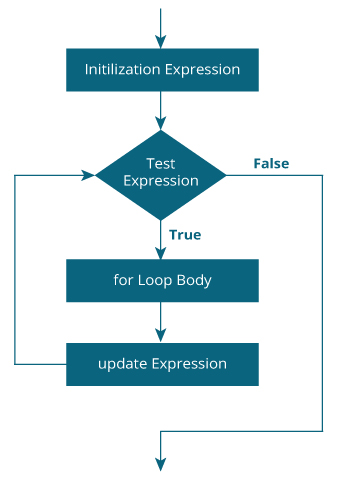### Looping Definition

Computers are often used to automate repetitive tasks. Repeating identical or similar tasks without making errors is something that computers do well and people do poorly.

Repeated execution of a set of statements is called iteration.

### Type of Loops in Python

Python has two statements for iteration

• While loop
• For loop

### The do-while Statement

Python doesn't support the do-while loop. But we can create a program like this.

The do while loop is used to check condition after executing the statement. It is similar to while loop but it is executed at last.

### Syntax:

``` do {
//statement
} while (condition);
```

### Example:

```i = 1
while True:
print(i)
i = i + 1
if(i > 5):
break
```

``` 1
2
3
4
5
```

### The while statement

The while loop in Python is used to iterate over a block of code as long as the test expression (condition) is true.

We enerally use this loop when we don't know beforehand, the number of times to iterate.

### The general syntax for the while loop:

``` while TEST_EXPRESSION:
STATEMENTS
```

In while loop, test expression is checked first. The body of the loop is entered only if the TEST_EXPRESSION evaluates to True. After one iteration, the test expression is checked again. This process continues until the TEST_EXPRESSION evaluates to False.

In Python, the body of the while loop is determined through indentation. Body starts with indentation and the first unindented line marks the end.

Python interprets any non-zero value as True. None and 0 are interpreted as False.

### Flowchart of while Loop### Example:

Python program to find sum of first n numbers using while loop

``` n = 5
sum = 0 # initialize sum and counter
i = 1
while i <= n:
sum = sum + i
i = i+1 # update counter
print("The sum is", sum) # print the sum
```

### Output:

``` The sum is 1
The sum is 3
The sum is 6
The sum is 10
The sum is 15
```

In the above program, the test expression will be True as long as our counter variable i is less than or equal to n (20 in our program).

We need to increase the value of counter variable in the body of the loop. This is very important (and mostly forgotten). Failing to do so will result in an infinite loop (never ending loop). Finally the result is displayed.

### The for Statement

The for loop in Python is used to iterate over a sequence (list, tuple, string) or other iterable objects. Iterating over a sequence is called traversal.

### The general syntax for the while stateme

``` for LOOP_VARIABLE in SEQUENCE:
STATEMENTS
```

Loop continues until we reach the last item in the sequence.

The body of for loop is separated from the rest of the code using indentation.

### Flowchart of for Loop### Example:

``` marks = [95,98,89,93,86]
total = 0
for subject_mark in marks:
total = total+subject_mark
print("Total Mark is ", total)
```

### Output:

``` Total Mark is  461
```

We can use the range() function in for loops to iterate through a sequence of numbers.

### Example:

``` sum=0
for i in range(5):
sum=sum+i
print("Sum is ", sum)
```

### Output:

``` Sum is  0
Sum is  1
Sum is  3
Sum is  6
Sum is  10
```